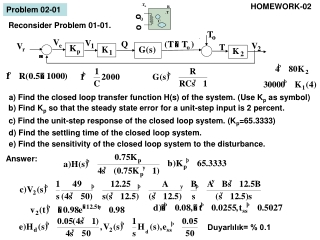DownloadDownload PresentationProblem 02-01

Problem 02-01

Download PresentationProblem 02-01

- - - - - - - - - - - - - - - - - - - - - - - - - - - E N D - - - - - - - - - - - - - - - - - - - - - - - - - - -
Presentation Transcript

1. HOMEWORK-02 o o Duyarlılık= % 0.1 Problem 02-01 Reconsider Problem 01-01. a) Find the closed loop transfer function H(s) of the system. (Use Kp as symbol) b) Find Kp so that the steady state error for a unit-step input is 2 percent. c) Find the unit-step response of the closed loop system. (Kp=65.3333) d) Find the settling time of the closed loop system. e) Find the sensitivity of the closed loop system to the disturbance. Answer:

2. Problem 02-02 (Kuo, p.214) Reconsider Problem 01-02. a) Find the open loop transfer function H1(s) of the system. b) Find the eigenvalues of open loop system. c) Find the closed loop transfer function H(s) of the system (Use Kp as symbol) d) Find the eigenvalues of the closed loop system.(Kp=9) e) Write the response’s form of the closed loop system for unit-step input. f) Find the steady state error. g) Find the damping ratio, the undamped frequency, the time step, the settling time for the closed loop system. Answer:

3. Problem 02-03 Reconsider Problem 01-03 a) Find the closed loop transfer function H(s) of the system. (Use Kp as symbol) b) Determine KP so that the sensitivity to disturbance is 4 percent. Answer:

4. Problem 02-04 Reconsider Problem 01-04. Write MatLAB programını that finds the eigenvalues of the closed loop system (Kp=1.8) Answer: clc;clear;kp=1.8; ra=1.15;jm=2120*1e-3*1e-4;bm=7*1e-2;ki=14.85*1e-2; kl=353.5;jl=3535*1e-3*1e-4;kb=15.5/1000/(2*pi/60); a11=[jl,0,kl];a12=-kl;a21=-kl*ra;a22=[jm*ra,bm*ra+ki*kb,kl*ra]; nh1=-a12;dh1=polyadd(conv(a11,a22),-conv(a12,a21)); nh=kp*nh1;dh=polyadd(dh1,kp*nh1);roots(dh)

5. a) Shaded area 1, s-düzlemi Problem 02-05 Kuo-91 (Sh.387) A closed loop control system’s eigenvalues requires to satisfy the following conditions. Determine the areas, that satisfies the criteria, in the s-plane. a) the overhoot < 6 %, The settling time < 0.8 s. Answer: S-plane

6. Problem 02-06 Kuo-91 (p.460) A unit-step response of a closed loop system is shown in the figure. Determine the prototype transfer function of the system. Yanıt: Problem 02-07 Kuo-91 (p.389) For the control system shown in the figure, find the values of K and Kt so that the maximum overshoot of the output is approximately 6 percent and the settling time is 1.5 s. Determine the steady-state error value for a unit-step input. Write a MATLAB program that finds the closed loop response to a unit-step input. Answer: